# 进程

## 进程模型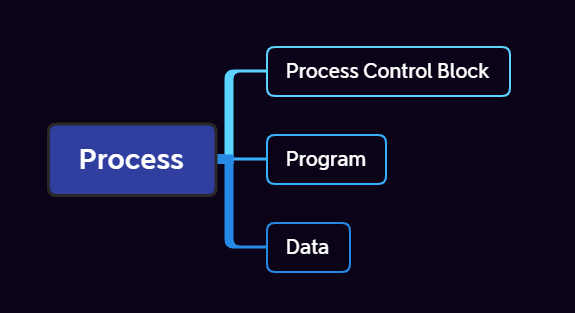Tips: The differences between process and program

Program is just the static text

Process is an dynamic entity being executed and has lifecycle

So if a program exec two times ,there should be two processes

## 进程的创建

1.系统初始化

2.正在运行的程序执行了创建进程的系统调用

3.用户请求创建一个新进程

4.批处理作业的初始化

fork后，创建的子进程会和父进程拥有相同的内存映像、环境变量。通常，子进程会接着执行一个execve之类的系统调用来修改内存映像从而运行一个新的程序。

## 进程的终止

1.正常退出(自愿的)

2.出错退出(自愿的) 自愿时通常调用exit系统调用

3.严重错误(非自愿)

4.被其他进程杀死(非自愿)//在Linux和Windows中，如果一个进程被杀死，但他创建的进程不会被全部杀死

## 进程的状态Running->Block：进程需要等待数据(自发)

## 进程的生命周期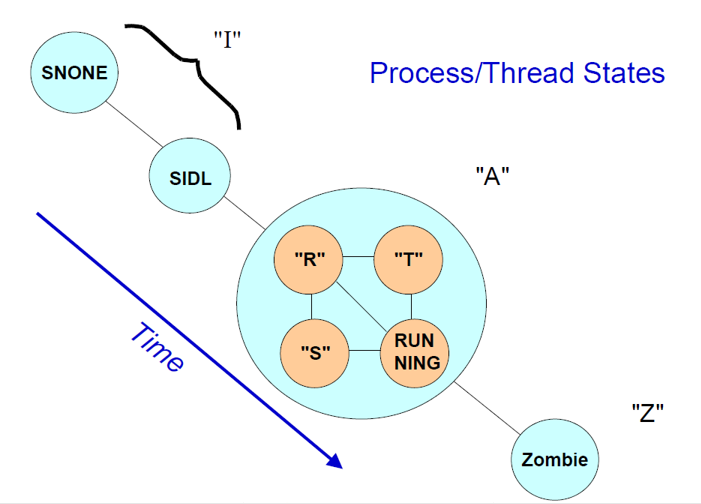I (Idle) state（空闲状态）

A (active) state（活跃状态）

Z (zombie) state（僵尸状态）

# 线程

## 经典线程、POSIX线程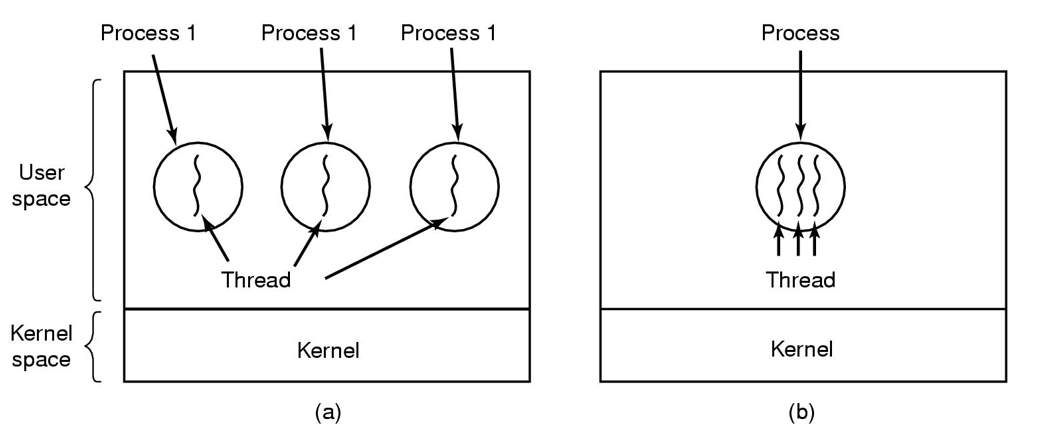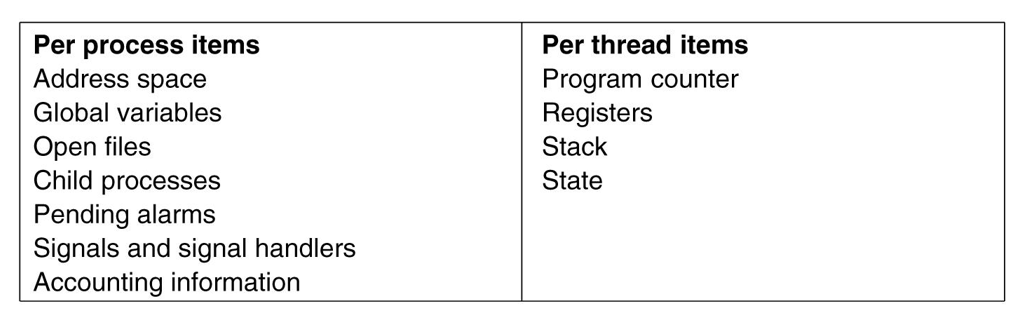POSIX线程

POSIX提供了很多系统调用来实现线程

``````#include <pthread.h>
#include <stdio.h>
#include <unistd.h>
#include <stdlib.h>
#include <time.h>

double PI;
long N;
double tim;

{
long id=(long)ID;
int i;
for(i=id*length;i<(id+1)*length;i++)
{
PI+=(4.0/N)*(1.0/(1+((i+0.5)/N)*((i+0.5)/N)));
}
}

int main()
{
printf("Please input the N of calculator\n");
scanf("%ld",&N);
clock_t start,end;
start=clock();
long i;
{
}
{
}
end=clock();
tim=(double)(end-start)/CLOCKS_PER_SEC;
printf("Running Time: %fs\n",tim);
printf("PI= %.15f\n",PI);
return 0;
}
``````

## 在用户/内核中实现线程

1.在用户空间实现线程

1.线程间切换很快

2.每个进程可以有自己的调度算法，且扩展性强

1.如何实现阻塞系统调用

2.starvation问题

3.最大问题出现在本身

# 调度程序(Important)

## 何时调度进程？

1.在创建一个新进程后，需要决定是运行父进程还是子进程

2.在一个进程退出时必须调度

3.进程阻塞时必须调度

4.一个IO中断发生时必须调度

## 调度算法分类及其目标

1.批处理(Batch System)

2.交互式(Interactive System)

3.实时(Real-time System)

## 批处理系统中的调度(Important)

### 先来先服务### 例题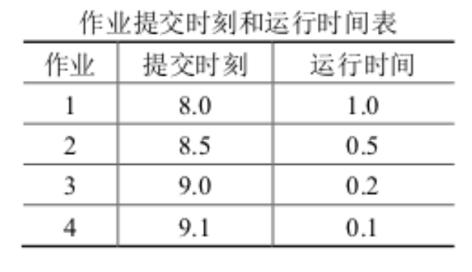1.先来先服务

1 8.0 1.0 8.0 9.0 1.0 1.0
2 8.5 0.5 9.0 9.5 1.0 2.0
3 9.0 0.2 9.5 9.7 0.7 3.5
4 9.1 0.1 9.7 9.8 0.7 7.0

2.短作业优先

1 8.0 1.0 8.0 9.0 1.0 1.0
3 9.0 0.2 9.0 9.2 0.2 1.0
4 9.1 0.1 9.2 9.3 0.2 2.0
2 8.5 0.5 9.3 9.8 1.3 2.6

3.高响应比优先

1 8.0 1.0 8.0 9.0 1.0 1.0
2 8.5 0.5 9.0 9.5 1.0 2.0
4 9.1 0.1 9.5 9.6 0.5 5.0
3 9.0 0.2 9.6 9.8 0.8 4.0

## 交互式系统中的调度(Important)

### Priority scheduling (优先级调度)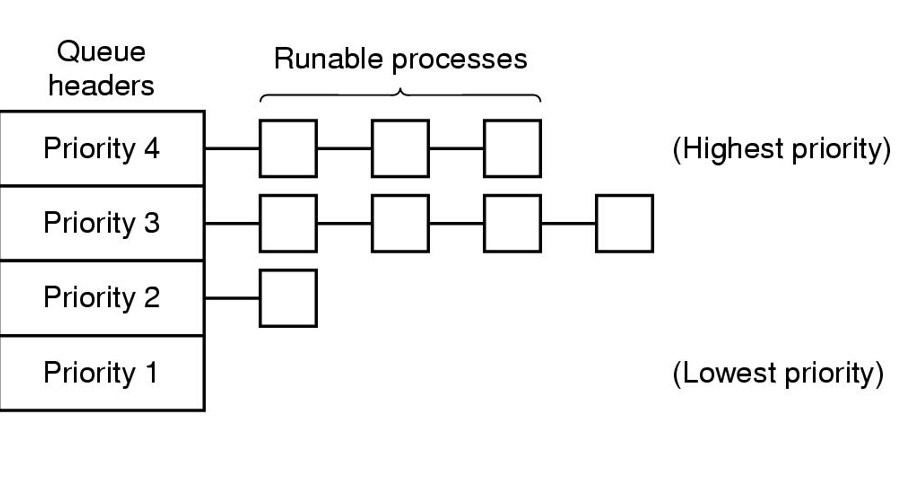# 进程间通信（Inter Process Communication, IPC）

## IPC的三个问题

1. 一个进程如何把信息传递给另一个。
2. 如何确保两个/多个进程在关键活动中不会出现交叉。（比如12306的购票系统）
3. 如何确保多个进程之间有正确的执行顺序（一个更改数据的操作和一个print操作，当数据更改后再print才是正确的）

## 基础概念（竞争条件&临界区）

All we need to do is 是两个进程不可能同时处于临界区。

1）任何两个进程不能同时在临界区中

2）不对CPU的速度和数量做任何假设

3）临界区外运行的进程不得阻塞其他进程

4）不得使进程无限期等待进入临界区

## 忙等待(busy waiting)的互斥

### 二、锁变量

So，这种方法只是转移了矛盾，并没有根本解决矛盾

### 三、严格轮换法

``````
int turn=0;
/*进程 0*/
while(true)
{
while(turn!=0)
critical_region();
turn=1;
noncritical_region();
}
/*进程 1*/
while(true)
{
while(turn!=1)
critical_region();
turn=0;
noncritical_region();
}``````

### 四、Peterson解法 (针对两个进程)

``````#define FALSE 0
#define TRUE 1
#define N 2 //进程数量

int turn; //轮到谁？

int interested[N]={0}; //所有值初始化为0

void enter_region(int process)/*进程号*/
{
int other;					    /*另一进程号*/
other=1-process;
interested[process]=TRUE;		/*表示感兴趣*/
turn = process;					/*设置标志*/
while(turn==process&&interested[other]==TRUE); /*空语句*/
}

void leave_region(int process) 		/*离开的进程*/
{
interested[process]=FALSE;		/*离开临界区*/
}``````

Attention：enter_region中的 turn 和 interest 设置顺序不能颠倒

``````void enter_region(int process)/*进程号*/
{
int other;					    /*另一进程号*/
other=1-process;
turn = process;					/*设置标志*/
interested[process]=TRUE;		/*表示感兴趣*/
while(turn==process&&interested[other]==TRUE); /*空语句*/
}``````

peterson算法的思想就是：类似孔融让梨，使用一个数组来表达每个进程想进入临界区的意图。每个进程要先表明自己的意图。最后一个进程要“让梨”，即如果turn是自己且对方想进入临界区，那自己就等待，让对方使用。

so…. 就出现了如此的尴尬局面。

### 五、TSL指令

``TSL RX, LOCK``

``````enter_region:
TSL REGISTER,LOCK		|复制锁到寄存器并将锁设为1
CMP REGISTER,\$0	 		|判断锁是否为0
JNE enter_region		|若非0，说明锁已被设置，进入busy waiting的循环
RET
leave_region:
MOVE LOCK,\$0			|锁中存0
RET				        |return``````

## 睡眠与唤醒（Sleep and wakeup）的互斥

peterson算法和TSL解法都是正确的，但他们都有忙等待的缺点——不仅会浪费时间，还会可能引起意外的结果。

sleep是一个引起调用进程阻塞的系统调用，即背挂起，直到被其他进程唤醒。

wakeup就是唤醒的进程咯QWQ！

code here ↓↓↓

``````#define N 100
int count=0;

void producer(void)
{
int item;
while(TRUE){
item=produce_item();
if(count==N)
sleep();
insert_item(item);
count=count+1;
if(count==1)
wakeup(consumer);
}
}

void consumer(void)
{
int item;
while(TRUE){
if(count==0)
sleep();
item=remove_item();
count=count-1;
if(count==N-1)
wakeup(producer);
consume_item(item);
}
}``````

emmm，他们就这样一起睡下去了。

## 信号量(Semaphore)的互斥与同步

PV操作是原子操作

P操作检查信号量，若大于0则将其减1。若等于0，则进程睡眠，且此时P操作并未结束。

V操作对信号量的值增加1,若一个或多个进程在该信号量上睡眠，则挑选一个允许完成其P操作。

PV在sleep wakeup的实现

``````/*P operation*/
P(Semaphore S)
{
S=S-1;
if(S<0){
added to the semaphore's queue and sleep;
}
}

/*V operation*/
V(Semaphore S)
{
S=S+1;
if(S<0){
wake up waiting process in the semaphore's queue;
}
}``````

PV在忙等待中的实现

``````/*P operation*/
P(Semaphore S)
{
while(!S>0)
yield the CPU;
S--;
}

/*V operation*/
V(Semaphore S)
{
S++;
}``````

``````#define N 100
typedef int semaphore;

semaphore mutex=1;
semaphore empty=N;
semaphore full=0;

void producer(void)
{
int item;
while(TRUE){
item=prodece_item();//产生数据
P(&empty);			//空槽--
P(&mutex);			//进入临界区
inster_item();		//数据放入临界区
V(&mutex);			//离开临界区
V(&full);			//满槽++
}
}

void consumer(void)
{
int item;
while(TRUE){
P(&full);		//满槽--
P(&mutex);		//进入临界区
item=remove_item();		//从缓冲区读出数据
V(&mutex);		//离开临界区
V(&empty);		//空槽++
consume_item(item);		//处理数据
}
}``````

## 哲学家就餐问题(同步)

``````#define N 5 /*哲学家数目*/
#define LEFT (i+N-1)%N /*i的左邻居编号*/
#define RIGHT (i+1)%N /*i的右邻居编号*/
#define THINKING 0 /*哲学家在思考*/
#define HUNGRY 1 /*哲学家试图拿起叉子*/
#define EATING 2 /*哲学家进餐*/

typedef int semaphore;

int state[N];/*记录每位哲学家的状态*/
semaphore mutex=1;/*临界区互斥*/
semaphore s[N];/*每个哲学家一个信号量*/

/*检查哲学家是否可以就餐*/
void test(int i/*每个哲学家编号*/)
{
if(state[i]==HUNGRY&&state[LEFT]!=EATING&&state[RIGHT]!=EATING){
state[i]=EATING;
V(&s[i]);
}
}
/*拿叉子，一次拿两个*/
void take_forks(int i)
{
P(&mutex);
state[i]=HUNGRY;/*记录哲学家i处于饥饿状态*/
test(i);	/*尝试获取两把叉子*/
V(&mutex);
P(&s[i]);	/*得不到需要的叉子就阻塞*/
}
/*放下叉子*/
void put_forks(int i)
{
P(&mutex);
state[i]=THINKING;/*哲学家就餐完毕*/
test(LEFT);
test(RIGHT);/*检查左右是否可吃*/
V(&mutex);
}

/*主函数*/
void philosopher(int i)
{
while(true){
think();
take_forks(i);/*获取两把叉子或阻塞*/
eat();
put_forks(i);/*放回两把叉子*/
}
}``````

## 读者—写者问题(互斥)

``````typedef int semaphore;
semaphore database=1;

{
while(true)
{
P(&mutex);
P(&database);
V(&mutex);
P(mutex);
V(&database);
V(&mutex);
use_data();
}
}

void writer(void)
{
while(true)
{
P(&database);//写者只关心数据库权限是否拥有即可。
write_data();
V(&database);
}
}``````

``````typedef int semaphore;
int rc=0;
int wc=0;
semaphore db=1;
semaphore rcmutex=1;
semaphore wcmutex=1;

{
P(&rcmutex);
if(rc==0) P(&db);
rc++;
V(&rcmutex);
P(&rcmutex);
rc--;
if(rc==0) V(&db);
V(&rcmutex);
}

void writer()
{
P(&wcmutex);
wc++;
V(&wcmutex);
P(&db);
write_data();
V(&db);
P(&wcmutex);
wc--;
V(&wcmutex);
}``````

``````typedef int semaphore;
int rc=0;
semaphore mutex=1;
semaphore db=1;
semaphore fair=1;

{
while(true){
P(&fair);
P(&mutex);
if(rc==0) P(&db);
rc++;
V(&mutex);
V(&fair);
P(&mutex);
rc--
if(rc==0) V(&db);
V(&mutex);
}
}

void writer()
{
while(true){
P(&fair);
P(&db);
write_data();
V(&db);
V(&fair);
}
}``````

# PV题

## 一、嗜睡的理发师

``````typedef int semaphore;
int waiting=0;
int chair=N;
semaphore barber=0,customer=0,mutex=1;

void Barber()
{
while(true){
P(customer);//无顾客，则睡眠
P(mutex);
waiting--;
V(mutex);
V(barber);//一个理发师开始理发
cut_hair();
}
}
void Customer()
{
while(true){
P(mutex);
if(waiting<chair){
waiting++;
V(mutex);
V(customer);//唤醒理发师
P(barber);//没人理发，就睡
get_hair_cut();//理发
}else{
V(mutex);
}

}
}``````

## 二、吸烟者问题

``````typedef int semaphore;
semaphore s=1;
semaphore s1=0,s2=0,s3=0;
bool material={0};

void supply()
{
while(true){
P(s);
int i=rand()%3;
material[(i+1)%3]=1;
material[(i+3-1)%3]=1;
if(material&&material)
{
V(s3);
}else if(material&&material){
V(s2);
}else{
V(s1);
}
}
}
void Smoker1()
{
while(true){
P(s1);
smoke();
V(s);
}
}
void Smoker2()
{
while(true){
P(s2);
somke();
V(s);
}
}
void Smoker3()
{
while(true){
P(s3);
somke();
V(s);
}
}``````

## 三、面包师问题

``````typedef int semaphore;
semaphore customer=0;
semaphore seller=n;
semaphore c_mutex=1;
semaphore s_mutex=1;
int number_c=0,number_s=0;

void Customer()
{
P(&c_mutex);
number_c++;
V(&c_mutex);
V(customer);
P(seller);
}

void Seller()
{
while(true){
P(&customer);
P(&s_mutex);
call the number_s customer;
number_s++;
V(&s_mutex);
V(&seller);
}

}``````

## 四、水果！

``````typedef int semaphore;
semaphore empty=0;
semaphore mutex=1;
semaphore apple=0;
semaphore orange=0;

{
while(true){
P(&empty);
P(&mutex);
put_fruit();
V(apple or orange);
V(&mutex);
}
}

void son()
{
while(true){
P(&orange);
P(&mutex);
get orange!
V(&mutex);
V(&empty);
}
}

void daugther()
{
while(true){
P(&apple);
P(&mutex);
get apple!
V(&mutex);
V(&empty);
}
}``````

## 五、仓库问题

（ 1）一次只能存入一种产品（ A 或 B）；
（ 2） -N < (A 产品数量-B 产品数量) < M。

``````typedef int semaphore;
semaphore mutex=1;
semaphore a=M-1;
semaphore b=N-1;

void putA()
{
P(&a);
P(&mutex);
PUT IN THE STORAGE!
V(&mutex);
V(&b);
}

void putB()
{
P(&b);
P(&mutex);
PUT IN THE STORAGE!
V(&mutex);
V(&a);
}``````

## 六、数字问题

``````typedef int semaphore;
semaphore mutex=1;
semaphore empty=N;
semaphore even=0;
semaphore odd=0;

void P1()
{
while(true){
int data=produce();
P(&empty);
P(mutex);
PUT DATA!
V(&mutex);
if(data%2==0)
V(&even);
else
V(&odd);
}
}
void P2()
{
while(true){
P(&odd);
P(&mutex);
getodd();
V(&mutex);
V(&empty);
countodd();
}
}

void P3()
{
while(true){
P(&even);
P(&mutex);
geteven();
V(&mutex);
V(&empty);
counteven();
}

}``````

## 七、窄桥问题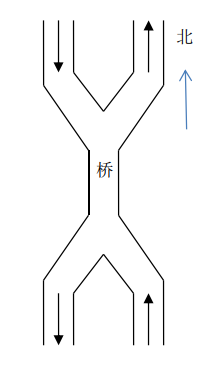``````typedef int semaphore;
semaphore bridge=1;
semaphore NS_mutex=1;
semaphore SN_mutex=1;

int NSC=0,SNC=0;

void N2S()
{
while(true){
P(&NS_mutex);
NSC++;
if(NSC==1) P(&bridge);
V(&NS_mutex);
GETTHROUGH!
P(&NS_mutex);
NSC--;
if(NSC==0) V(&bridge);
V(&NS_mutex);
}
}

void S2N()
{
while(true){
P(&SN_mutex);
SNC++;
if(SNC==1) P(&bridge);
V(&SN_mutex);
GETTHROUGH!
P(NS_mutex);
SNC--;
if(SNC==0) V(&bridge);
V(&NS_mutex)；
}
}``````